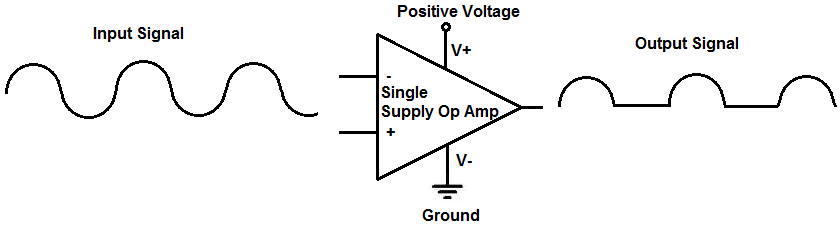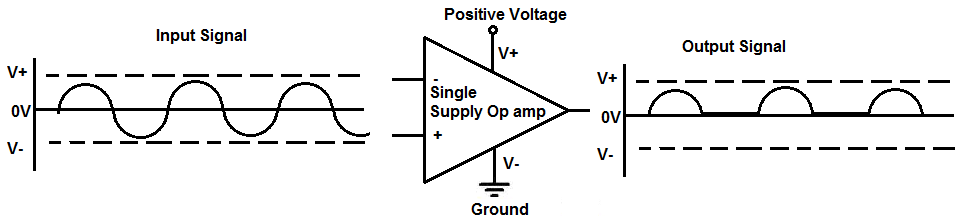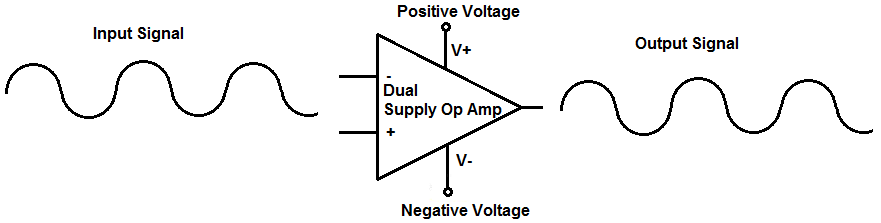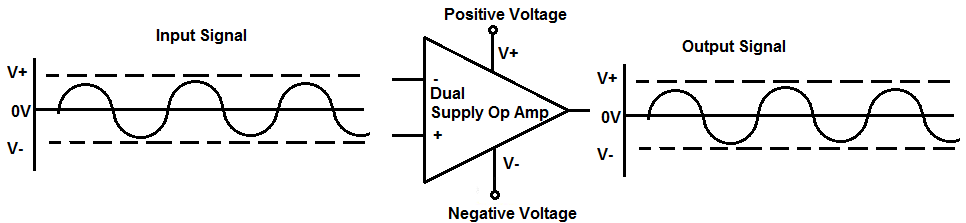﻿ What is the Difference Between a Single and Dual Supply Op Amp? ﻿# What is the Difference Between a Single and Dual Supply Op Amp?In this article, we explain the difference between a single and dual supply op amp.

Obviously, both are op amps.

The difference between the two is that a single supply op amp receives a single voltage while a dual supply op amp receives 2 voltages, a positive and negative voltage.

The difference is pretty pronounce.

With a single supply op amp, the V+ terminal of the op amp receives a positive voltage and the V- terminal connects to ground. A signal into the op amp can only swing as far as the power supply allows. Therefore, any input signal fed into the op amp can only swing from the positive voltage supply to ground.

With a dual supply op amp, the V+ terminal of the op receives a positive voltage and the V- terminal connects to negative voltage. Therefore, any input signal fed into the op amp can swing from the positive voltage supply to the negative voltage supply.

Therefore, single supply op amps really don't work well with AC signals, because if an AC signal is fed into a single supply op amp, it can swing from the positive voltage to ground. Therefore, the whole negative portion of the AC signal gets clipped. This is why single supply op amps don't lend well in dealing with full cycles of AC signals. The exception is if you using the op amp as a half wave rectifier. However, if you are dealing with the full cycles of AC signals, single supply op amps don't work well with them.

Dual supply op amps, on the other hand, are much more versatile in that they allow signals to swing a greater distance. Therefore, unlike a single supply op amp, they can swing all the way from the positive voltage to the negative voltage, covering the full cycle, positive and negative portions of the AC signal.

We will now illustrate the single and dual op amps and how the output voltage is different depending on which is used.

### Single Supply Op Amp

So below is a diagram of a single supply op amp and an AC input voltage fed into it and the resulting output voltage waveform.So the diagram above is of a single supply op amp.

The input voltage fed into this op amp is an AC signal that swings from positive voltage to the 0V line and then to negative voltage. This cycle repeats over and over again.

The resulting output signal is the AC waveform with the waveform clipped at the negative portion. The AC signal never swings negative at the output, because the power supply of the op amp is positive voltage fed into V+ and V- connected to ground. Therefore, the AC signal fed into the single supply op amp can only swing as high as the DC voltage fed into V+ and goes as low as GND. The DC voltage fed into V+ and V- forms the rails of the output AC voltage signal.

Therefore, for example, if we feed +15V into V+ and V- is connected to ground, the AC signal can swing as high as to +15V and goes down to as low as ground.

If we fed +20V into V+ and V- is connected to ground, the AC signal can swing as high as to +20V and goes down as low as to ground.

So you can see now how the rails are set for an AC signal.

The AC signal can never swing negative with a single supply op amp because the floor is set to ground. It isn't set to a negative voltage like a dual supply op amp, so it can't go negative. It simply cuts off at its floor, which is ground.

A more in depth breakdown of the signal analysis is shown in the diagram below.The diagram before this was simpler, but this diagram gives the exact breakdown of the input and output signal.

You can see specifically how the input AC signal swings from positive to negative voltage, crossing the 0V line in between.

At the output, the AC signal is exactly traced at the positive portion of the AC signal. As the signal descends, it goes only to the 0V line, which is ground. The signal cannot go to negative, because the V- line is grounded, not set to a negative voltage. So the negative portion of the signal is entirely clipped. The signal remains at the 0V line at the entirety of the negative portion of the signal.

This is how AC signals work with single supply op amps.

Thus, battery-operated and other DC-power-operated devices are frequently used with single supply op amps.

Examples of single supply op amps are the AD820 and LT1078 op amps.

### Dual Supply Op Amps

So now we go to dual supply op amps.

Dual supply op amps really have 2 power supplies, a positive voltage fed to V+ and a negative voltage fed to V-.

The difference for dual supply op amps is that it can swing positive all the way to negative, capturing the entire AC signal cycle.

Below is the diagram of a dual supply op amp when fed an AC signal.So the AC signal fed into the dual supply op amp goes from positive to negative, crossing the 0V line in between.

The signal at the output is the exact mirror of this signal. Unlike a single supply op amp, the dual supply op amp is able to swing from positive all the way down to negative, because the op amp has a positive and negative rail, set by the DC voltages fed into V+ and V-.

With a dual supply op amp, the output AC signal is able to swing as high as V+ and as low as V-. Unlike a sinlge supply op amp which is connected to ground and cuts off at the 0V line, a dual supply op amp can go negative, as far negative as the negative voltage fed into V-.

So the dual supply op amp has a positive rail and a negative rail.

The single supply op amp just has a positive rail and ground, restricting the AC signal from V+ to ground.

The dual supply op amp can swing from V+ to V-.

So, for example, if we feed +15V into V+ and -15V into V-, the op amp accomodate a signal that is 30V peak-to-peak; that is 15V positive and 15V negative. This of course is assuming that the op amp can handle such voltages.

As another example, if we feed +20V into V+ and -20V into V-, the op amp can accomodate a signal that is 40V peak-to-peak; that is 20V positive and 20V negative.

A more in depth breakdown of the signal analysis is shown in the diagram below.You can see how the input and output signals are mirrors of each other. Being that the dual supply op amps allows for the positive and negative portions of AC signals, the full AC signal is reproduced at the output.

For op amps, be careful to make sure that the rails you want to set for your op amp is within the power constraints of the op amp in use .For example, if you are using an LM741, a very popular operational amplifier, the maximum voltage it can deal with is ±22V. Therefore, the maximum voltage peak to peak it can deal with is 44V peak to peak. The point is to make sure that the op amp can handle the rails you set. If the op amp can only withstand 20V, and you want to set a 25V rail, it isn't possible due to the constraints of the op amp. So, in that case, you would have to use an op amp that has larger constraints.

Another thing that should also be put into consideration is the slew rate of the op amp. The slew rate is the rate at which an op amp can output voltage per unit time. The size of the signal and the frequency of the signal determine how fast an op amp can put out voltage. With a very large voltage (high amplitude) and a fast frequency, the op amp may not be able to output the signal quick enough if it has a low enough slew rate. Therefore, this is also another major consideration when dealing with op amp circuits.

But looking at the differences between single and dual supply op amps, dual supply are more apt to working with AC signals while dealing with DC signals or half wave rectifiers work well with dealing with single supply op amps.

Related Resources